# Simon’s Randomized Phase II Design

Data Input: (Help) (Example)

 Input Results p D n k Prob

Calc A: To calculate “n” until the Prob is at least 0.9

Calc B: To calculate “Prob” with your input “n”

*Since the calculation takes some times, if the Web Browser shows the pop-up window to ask you to stop the script, please click "No" until the results have shown.

Note:

 Variables Descriptions p Lowest response rate among all treatments D Difference in response rate between the best treatment and the other treatments k Number of treatment arms n Number of patients in each treatment arm Prob Probability of correctly selecting the best treatment

Help Aids

Application:  In phase II clinical trial, randomized design is proposed to establish the sample size for the study to obtain the treatment with greatest response rate for further / phase III study.

Procedure:

1. Enter

a)   value of p, the lowest response rate among all treatments

b)   value of D, the difference between the best treatment and the other treatments

c)   value of k, the number of treatment arms

if click the button “Calc B” next step, go 1 (d) and then step 3, otherwise go step 2 only

d)   value of n, the number of patients in each treatment arm

1. Click the button “Calc A” to obtain

a)    value of n, the number of patients in each treatment arm

b)    value of Prob, the probability of correctly selecting the best treatment.

1. Click the button “Calc B” to obtain

a)    value of Prob, the probability of correctly selecting the best treatment.

Theory:

The treatment selected is that with the largest observed response rate. Foe selecting among K treatments when the difference in true response rates of the best and next best treatment is D, the probability of correct selection is smallest when there is a single best treatment and the other K-1 treatments are of equal but lower efficacy. If we stipulate that the response rate of the worst treatment equals a specified value p, then the probability that the best treatment produces the highest observed response rate is: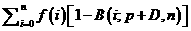(1)

where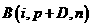is the cumulative distribution function for a binomial distribution with success parameter p+D and n patients and f(i) is the probability that the maximum number of success observed among K-1 inferior treatment is i. Thus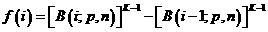If there is a tie among the treatments for the largest observed response rate, we shall assume that one of the tied treatments is randomly selected. Hence, in calculating the probability of correct selection, we add to expression (1) the probability that the best treatment was selected after being tied with 1 or more of the other treatments for the greatest observed response rate. This probability is: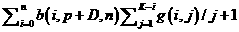(2)

where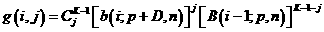, b denotes the binomial probability mass function. The quantity g(i,j) represents the probability that exact j of the inferior treatments are tied for the largest number of observed responses among the K-1 inferior treatments, and this number of responses is i. The factor 1/(j+1) in expression (2) is the probability that tie among the best treatment and the j inferior treatments is randomly broken by selecting the best treatment.

The probability of correct selection is the sum of expressions (1) and (2). For specified values of p, D and K, the value of n, number of patients per treatment, is determined to provide a probability of correct selection equal to that desired (P).

Discussion:

There are some advantages to the randomized Phase II design of two or more new agents.

1.      Randomization helps ensure that patients are centrally registered before treatment starts. Establishment of a reliable mechanism to ensure patient registration prior to treatment is of fundamental importance for all clinical trials.

2.      Comparing to independent phase II studies, the differences in results obtained for the two agents will more likely represent real differences in toxicity or antitumor effects rather than differences in patient selection, response evaluation, or other factors.

Example:

Suppose that three schedules (k=3) of administration are to be studied and the expected baseline response rate is 20% (p=0.2). With 44 (n=44) patients per schedule, we have probability 0.9 (Prob=0.90) of selecting the schedule that has a true response rate of 20%+15%=35% (D=0.15).

Reference: Simon, Wittes and Ellenberg (1985) Cancer Treatment Reports 69: 1375-1381